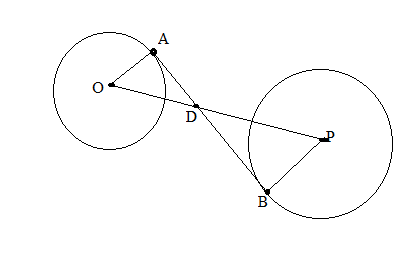Chapter 6.3, Problem 38E### Elementary Geometry for College St...

6th Edition
Daniel C. Alexander + 1 other
ISBN: 9781285195698

#### Solutions

Chapter
Section### Elementary Geometry for College St...

6th Edition
Daniel C. Alexander + 1 other
ISBN: 9781285195698
Textbook Problem
5 views

# The center of a circle of radius 3 inches is at a distance of 20 inches from the center of a circle of radius 9 inches. What is the exact length of common internal tangent A B ¯ ?(HINT: Use similar triangles to find OD and DP. Then apply the Pythagorean Theorem twice.)To determine

To find:

The find value of AB¯.

Explanation

Given that, the center of a circle of radius 3 in. is at a distance of 20 in. from the center of a circle of radius 9 in.

That is OA=3,PB=9 and OP=20 then AB¯ is common internal tangent.

The diagrammatic representation is given below,

Since AB¯ is common internal tangent therefore, AB¯OA¯ and AB¯PB¯

Using the vertical angle theorem to get the following,

If two triangles are similar, then the ration of any two corresponding segments (such as altitudes, medians, or angle bisectors) equals the ratio of any two corresponding sides.

OA¯PB¯=OD¯PD¯39=OD¯PD¯OD¯PD¯=133OD¯=PD¯

We know that,

OP¯=OD¯+PD¯20=OD¯+PD¯PD¯=20OD¯. Since OP¯=20

Substitute this in the above equation to get the following,

### Still sussing out bartleby?

Check out a sample textbook solution.

See a sample solution

#### The Solution to Your Study Problems

Bartleby provides explanations to thousands of textbook problems written by our experts, many with advanced degrees!

Get Started

#### Simplify the expressions in Exercises 97106. (xy)1/3(yx)1/3

Finite Mathematics and Applied Calculus (MindTap Course List)

#### In Exercises 13-20, sketch a set of coordinate axes and plot each point. 20. (1.2, 3.4)

Applied Calculus for the Managerial, Life, and Social Sciences: A Brief Approach

#### Sometimes, Always, or Never: (ax) = ax.

Study Guide for Stewart's Single Variable Calculus: Early Transcendentals, 8th